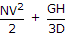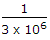# Electronics and Communication Engineering - Microprocessors

### Exercise :: Microprocessors - Section 3

1.

Consider the following rules about integer constant in C

1. An integer constant must have at least one digit.
2. An integer constant must not have a decimal point.
3. An integer constant may be positive or negative.
4. The default sign is positive.
5. A maximum of one comma or blank is allowed.
Which of the above are correct?

 A. All B. 1, 2, 3, 4 only C. 1, 2, 3, 5 D. 2, 3, 4, 5 only

Explanation:

No comma or blank is allowed in integer constant in C.

2.

Consider the following logical IF statement in Fortran 77 `IF (SALT. LE. PEPPER) 11GO TO 13` The above statement using arithmetic IF statement would be

 A. IF (SALT - PEPPER) 11, 11, 13 B. IF (SALT - PEPPER) 13, 11, 11 C. IF (SALT - PEPPER) 13, 13, 11 D. IF (SALT - PEPPER) 11, 13, 13

Explanation:

The logical IF statement means that IF SALT is less than or equal to PEPPER, control goes to statement 11 otherwise (i.e., SALT greater than PEPPER), control goes to 13 Same is true of arithmetic IF statement (a).

3.

Consider the following rules for constructing variable names in C

1. It can have alphabets, digits or underscores but no other special character.
2. The maximum number of characters is 8.
3. The first character may be an alphabet or digit but not underscore.
Which of the above are correct?

 A. All B. 1 and 2 only C. 1 and 3 only D. 2 and 3 only

Explanation:

First character must be alphabet only.

4.

The correct way of writing the expressionin Pascal is

 A. `N * V * V / 2 + G * H / 3D` B. `N * V * V / 2.0 + G * H / (3.0 *D)` C. `N * V * V / 2 + G * H / 3.0 * D` D. `N * V * V / 2.0 + G * H / 3.0 * D`

Explanation:

Operators must be written. They are not implied.

5.

A microprocessor uses 3 MHz oscillator. The duration of one T state is

 A. 1 μs B. 0.666 μs C. 0.333 μs D. 3 μs= 0.333 x 10-6 s.